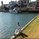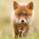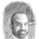3472 views
Murrey's Math Lines Box, top and bottom values of the final box must be entered manually.
```study(shorttitle="[RS]MMLB", title="[RS]Murreys Math Lines Box", overlay=true)
btop = input(147.66, type=float)
bbot = input(75.555, type=float)

fraction = (btop - bbot) * 0.125

l00 = hline(btop + (fraction * 2), color=black, linewidth=1, title="")
l01 = hline(btop + (fraction * 1), color=black, linewidth=1, title="")
l02 = hline(btop, color=black, linewidth=3, title="8/8: Hardest line to rise above (overbought)")
l03 = hline(btop - (fraction * 1), color=black, linewidth=1, title="7/8: Fast reverse line (weak)")
l04 = hline(btop - (fraction * 2), color=black, linewidth=1, title="6/8: Pivot reverse line")
l05 = hline(btop - (fraction * 3), color=black, linewidth=1, title="5/8: Upper trading range")
l06 = hline(bbot + (fraction * 4), color=black, linewidth=2, title="4/8: Major reversal line")
l07 = hline(bbot + (fraction * 3), color=black, linewidth=1, title="3/8: Lower trading range")
l08 = hline(bbot + (fraction * 2), color=black, linewidth=1, title="2/8: Pivot reverse line")
l09 = hline(bbot + (fraction * 1), color=black, linewidth=1, title="1/8: Fast reverse line (weak)")
l10 = hline(bbot, color=black, linewidth=3, title="0/8: Hardest line to fall below (oversold)")
l11 = hline(bbot - (fraction * 1), color=black, linewidth=1, title="")
l12 = hline(bbot - (fraction * 2), color=black, linewidth=1, title="")

plot(close==0? na : na)
```GREAT WORK MATE.. AWESOME... WORKS LIKE A HOT KNIFE ON COLD BUTTER.. THANNNKKKSSSSS..